PHY294H - Lecture 5

Recommended Problems for Lecture 5: FGT: 24.25, 24.26, 24.31, 24.47
Recommended Reading for lecture 6: FGT Ch. 24

Coulomb's law, again!!

For the next few days we shall look at Coulomb's law in in a new way. In fact, this new way of looking at electrostatics is so important it is given a name of its own, it is called Gauss' law. Gauss' law is a method for calculating the electric field produced by charges, which are considered the sources of the electric field. We will see that sometimes Gauss' law is a much more efficient way of calculating the electric field. Usually if you can see a way of finding the electric field using Gauss' law, do it. It is almost always easier than doing the vector sums or integrals that we have gone through so far. We start by stating Gauss' law and then we shall go through the various terms in this equation. Gauss' law is,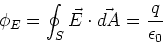(1)

-is the electric flux

- S is a closed surface (we can choose this to suit ourselves)

-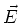is the electric field

-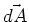is a surface element. Its direction,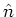is normal to the surface at that point. We shall often write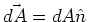.

- q is the sum of all the charges that lie inside the closed surface S.

What is flux?

You are used to flux in the case of current or fluid flow. The flux through a surface A is just the total amount of fluid or current that flows through that surface. Notice that it is possible to draw may different surfaces A that cross a flow, and the total flow through that surface is the same. The total current across a surface A is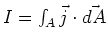.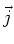is the current density. We have a lot of freedom in choosing the surface that we use and this is often extremely useful. The concept of flux also applies to many problems where field lines can be defined. The concept of electric flux is direction analogous to the concept of current, but we only replace the current density by the electric field. Later in the course, we shall also define a magnetic field and its associated magnetic flux. Similarly it is possible to define a flux associated with the gravitational field.

We can take this analogy a bit further. If we have a set of point sources of current, then the amount of flow (flux) through a surface surrounding these point sources should be equal to the current coming out of the sources (e.g. mountain springs). Gauss' law says the same thing. The charges in EM are sources of the electric field so any surface enclosing a set of charges should have a total flux proportional to the amount of charge enclosed by the surface.

Using Gauss' law

A point charge, Q

Whenever we use Gauss' law, the first thing we need to do is choose a Gaussian surface. In this case we choose the closed surface S = surface of a sphere, centered at the point charge. The area of the surface of a sphere is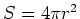.

- The surface normal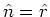, a unit vector in the radial direction.

- q, the enclosed charge, is just Q the point charge.

- By symmetry, the direction of the electric field is the radial direction, so we write,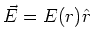, where E(r) is the magnitude of the electric field at radial distance r from the point charge.

The electric flux is,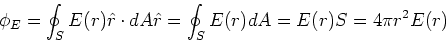(2)

Gauss' law then gives,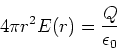(3)

and we find,(4)

Which is, of course, the electric field due to a point charge. Notice that Gauss's law implies that Coulomb's law is just a conservation law for the number of field lines coming from a charge. This is very appealing from a physical point of view.

A uniform sheet of charge

Another problem to which we know the answer already is a uniform sheet of charge. We consider a thin, flat, sheet with uniform charge density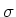, which we take to be positive, and which lies in the x-y plane at z=0.

- Choose the Gaussian surface to be a thin slab. The surface of this thin slab is composed of six planes. Two planes of area Aa each lie at z=a and at z=-a. The surface normals to these planes are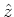and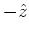respectively. There are also two planes atwith area Ab, which have surface normals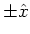respectively, and two planes at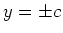with area Ac, with surface normals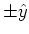.

- By symmetry the direction of the electric field isabove the charged sheet andbelow the charged sheet.

- The enclosed charge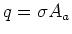.

The electric flux through the Gaussian surface is,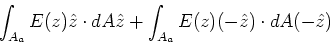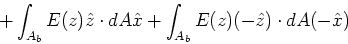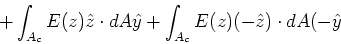(5)

This has been written out in all of its gory detail, though many of you would have immediately seen that the areas Ab and Ac give zero contribution because the electric field and the surface normal are perpendicular. In physical terms, there is no flux through these surfaces because no field lines cross these surfaces. From the above expression, we have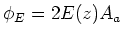. Gauss's law then gives,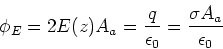(6)

so that the electric field due to a uniform sheet of charge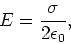(7)

as we found before. As can be seen from the above, the analysis using Gauss' law is algebraically simple but conceptually sophisticated.

Electric field near a conductoring slab

Consider a conducting slab with uniform charge densityat its surface. Construct a Gaussian surface which is also a slab, but now with one of the areas Aa of the example above lying inside the conductor. All of the arguments of the above example hold, except that the surface Aa inside the conductor has. It is then evident that the electric field at the surface of the conductor is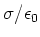.

Shell theorems

Consider a thin spherical shell of radius, R, and total charge Q. Recall that the shell theorems are:

- There is no electric field inside a uniform spherical shell of charge

- The electric field outside a uniform spherical shell of total charge Q is like that of a point charge Q at the center of the shell.

Now that we have Gauss' law, the above shell theorems can be proven very straightforwardly. Consider the Gaussian surface to be a spherical shell of radius r, with surface area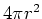and surface normal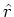. The electric field is in thedirection, by symmetry. The electric flux is then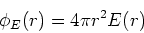(8)

which is exactly the same as for a point charge. Now consider the two cases:

- r<R. In that case the enclosed charge is zero, q=0. This implies that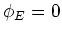, which in turn implies that E(r) = 0.

- r>R. In that case, the enclosed charge is Q the charge of the spherical shell. Then the electric field is found from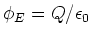, which gives the expression for a point charge Q at the center of the spherical shell.

Gauss' law for gravity

If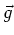is the gravitational field, then Gauss' law for gravity is,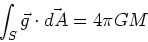(9)

where M is the total mass inside the Gaussian surface.